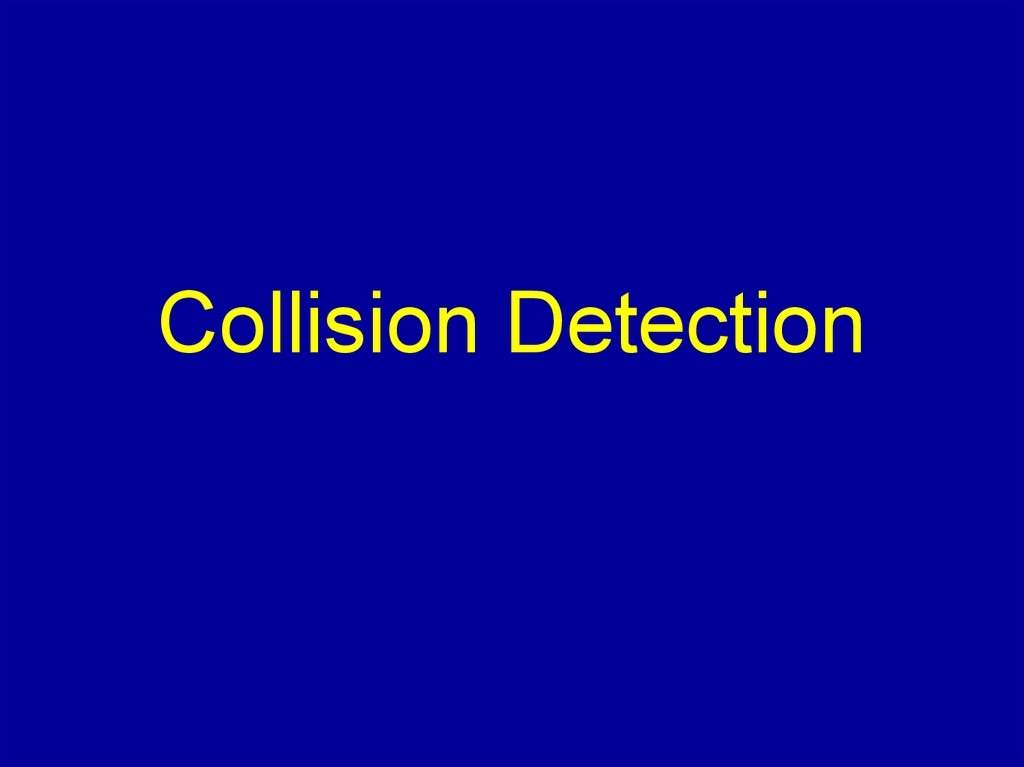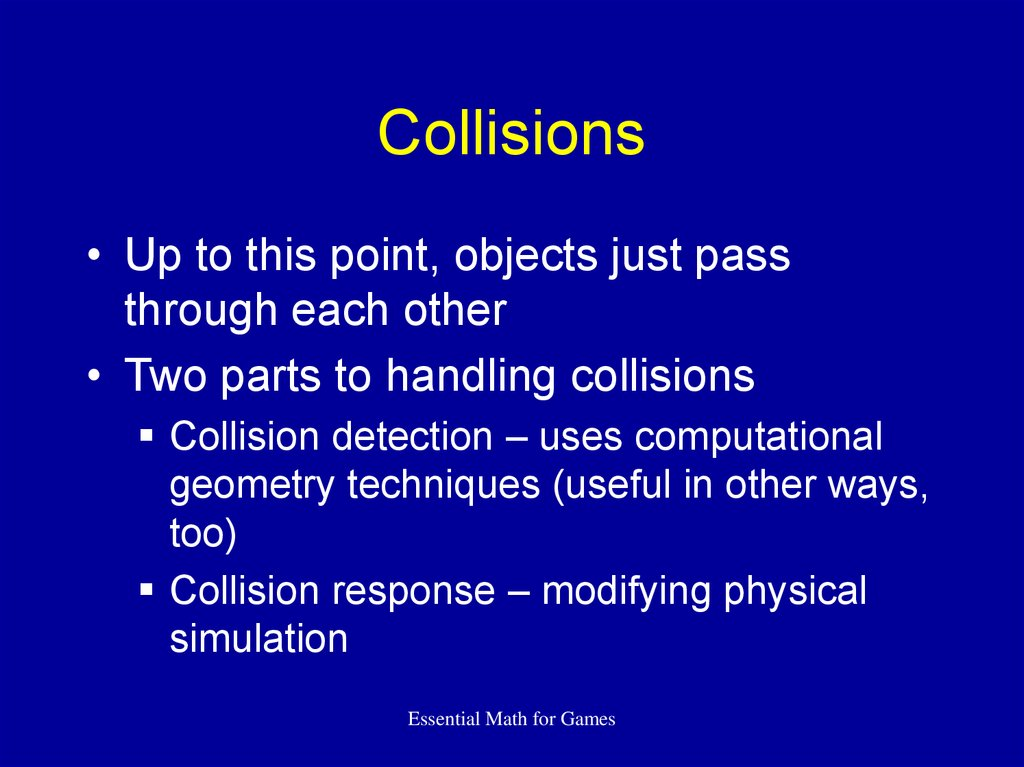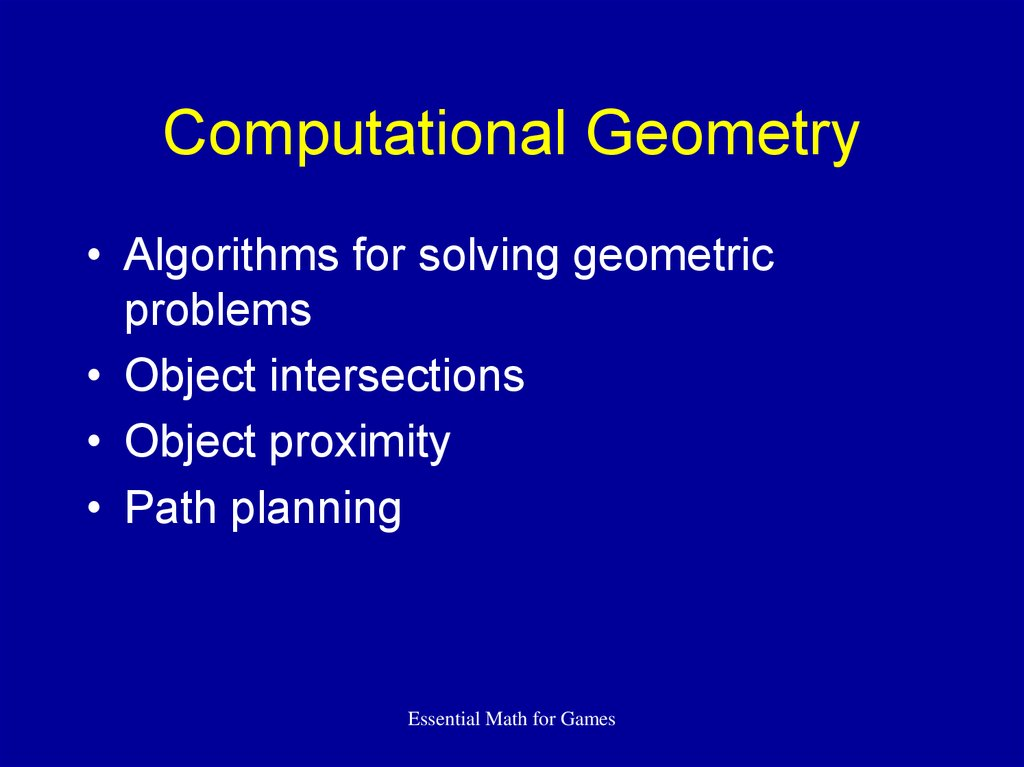# Collision Detection

## 2. Collisions

• Up to this point, objects just pass
through each other
• Two parts to handling collisions
Collision detection – uses computational
geometry techniques (useful in other ways,
too)
Collision response – modifying physical
simulation
Essential Math for Games

## 3. Computational Geometry

• Algorithms for solving geometric
problems
• Object intersections
• Object proximity
• Path planning
Essential Math for Games

## 4. Distance Testing

• Useful for computing intersection
between simple objects
• E.g. sphere intersection boils down to
point-point distance test
• Just cover a few examples
Essential Math for Games

## 5. Point-Point Distance

• Compute length of vector between two
points P0 and P1, or
Essential Math for Games

## 6. Line-Point Distance

Line defined by point P and vector ^
v
Break vector w = Q – P into w and w||
w|| = (w ^v) ^v
Q
||w ||2 = ||w||2 – ||w||||2
w
w
w||
P
^
v
Essential Math for Games

## 7. Line-Point Distance

• Final formula:
• If v isn't normalized:
Essential Math for Games

## 8. Line-Line Distance

• From http://www.geometryalgorithms.com
• Vector wc perpendicular to u and v or
P0
u
P(sc)
v
wc
• Two equations
• Two unknowns
Essential Math for Games
Q(tc)
Q0

## 9. Line-Line Distance

Final equations:
P0
u
P(sc)
v
Q(tc)
Q0
Essential Math for Games

## 10. Segment-Segment Distance

• Determine closest point between lines
• If lies on both segments, done
• Otherwise clamp against nearest
endpoint and recompute
• See references for details
Essential Math for Games

## 11. Bounding Objects

• Detecting intersections with complex
objects expensive
• Provide simple object that surrounds
them to cheaply cull out obvious cases
• Use for collision, rendering, picking
• Cover in increasing order of complexity
Essential Math for Games

## 12. Bounding Sphere

• Tightest sphere that surrounds model
• For each point, compute distance from
Essential Math for Games

## 13. Bounding Sphere (Cont’d)

• What to use for center?
Local origin of model
Centroid (average of all points)
Center of bounding box
• Want a good fit to cull as much as
possible
• Linear programming gives smallest fit
Essential Math for Games

## 14. Sphere-Sphere Collision

• Compute distance d between centers
• If d < r1 + r2, colliding
• Note: d2 is not necessarily < r12 + r22
want d2 < (r1 + r2)2
r2
d
r1
Essential Math for Games

## 15. Bounding Box

• Tightest box that surrounds model
• Compare points to min/max vertices
• If element less/greater, set element in
min/max
(max x, max y)
(min x, min y)
Essential Math for Games

## 16. Axis-Aligned Bounding Box

• Box edges aligned to world axes
• Recalc when object changes orientation
• Collision checks are cheaper though
Essential Math for Games

## 17. Axis-Aligned Box-Box Collision

• Compare x values in min,max vertices
• If min2 > max1 or min1 > max2, no
collision (separating plane)
min1
max1
min2
max2
• Otherwise check y and z directions
Essential Math for Games

## 18. Object-Oriented Bounding Box

• Box edges aligned with local object
coordinate system
• Much tighter, but collision calcs costly
Essential Math for Games

## 19. OBB Collision

• Idea: determine if separating plane
between boxes exists
• Project box extent onto plane vector,
test against projection btwn centers
c
a
b
b v
a v
c v
Essential Math for Games

## 20. OBB Collision

• To ensure maximum extents, take dot
product using only absolute values
• Check against axes for both boxes, plus
cross products of all axes
• See Gottschalk for more details
Essential Math for Games

## 21. Capsule

• Cylinder with hemispheres on ends
• One way to compute
Calc bounding box
Use long axis for length
r
Essential Math for Games
r

## 22. Capsule

• Compact
Only store radius, endpoints of line
segment
• Oriented shape w/faster test than OBB
• Test path collision
Essential Math for Games

## 23. Capsule-Capsule Collision

• Key: swept sphere axis is line segment
• Compute distance between line
segments
• If less than r1 + r2, collide
Essential Math for Games

## 24. Caveat

Math assumes infinite precision
Floating point is not to be trusted
Precision worse farther from 0
Use epsilons
Careful of operation order
Re-use computed results
More on floating point on website
Essential Math for Games

## 25. Which To Use?

• As many as necessary
Sphere
Swept Sphere
Box
• May not need them all
Essential Math for Games

## 26. Recap

• Sphere -- cheap, not a good fit
• AABB -- still cheap, but must recalc and
not a tight fit
• Swept Sphere -- oriented, cheaper than
OBB but generally not as good a fit
• OBB -- somewhat costly, but a better fit
Essential Math for Games

## 27. Collision Detection

• Naïve: n2 checks!
• Two part process
• Cull out non-colliding pairs
Narrow phase
• Determine penetration and contact points
between pairs
Essential Math for Games

• Obvious steps
Only check each pair once
• Flag object if collisions already checked
Only check moving objects
• Check against other moving and static
Check rough bounding object first
• AABB or sphere
Essential Math for Games

## 29. Hierarchical Systems

• Can break model into hierarchy and
build bounds for each level of hierarchy
• Finer level of detection
• Test top level, cull out lots of lower
levels
Essential Math for Games

## 30. Hierarchical Systems

• Can use scene graph to maintain
bounding information
• Propagate transforms down to children
• Propagate bound changes up to root
Essential Math for Games

## 31. Spatial Subdivision

• Break world into separate areas
• Only check your area and neighbors
• Simplest: uniform
Slabs
Grid
Voxels
Essential Math for Games

## 32. Sweep and Prune

• Store sorted x extents of objects
• Sweep from min x to max x
• As object min value comes up, make
active, test against active objects
• Can extend to more dimensions
Essential Math for Games

## 33. Spatial Subdivision

• Other methods:
BSP trees, kd-trees
Room-portal
• Choice depends on your game type,
rendering engine, memory available,
etc.
Essential Math for Games

## 34. Temporal Coherence

• Objects nearby generally stay nearby
• Check those first
• Can take memory to store information
Essential Math for Games

## 35. Narrow Phase

• Have culled object pairs
• Need to find
Contact point
Normal
Penetration (if any)
Essential Math for Games

## 36. Contact Region

• Two objects interpenetrate, have one
(or more) regions
• A bit messy to deal with
• Many try to avoid interpenetration
Essential Math for Games

## 37. Contact Features

• Faceted objects collide at pair of contact
features
• Only consider E-E and F-V pairs
• Infinite possibilities for normals for
others
• Can generally convert to E-E and F-V
• Ex: V-V, pick neighboring face for one
Essential Math for Games

## 38. Contact Features

• For E-E:
Point is intersection of edges
Normal is cross product of edge vectors
• For F-V:
Point is vertex location
Normal is face normal
Essential Math for Games

## 39. Contact Points

• Can have multiple contact points
Ex: two concave objects
• Store as part of collision detection
• Collate as part of collision resolution
Essential Math for Games

## 40. Example: Spheres

• Difference between centers gives
normal n (after you normalize)
c1
c2
• Penetration distance p is
p = (r1+r2) - ||c2-c1||
Essential Math for Games

## 41. Example: Spheres

• Collision point: average of penetration
distance along extended normal
^
v = ½(c1 + r1n^ + c2 - r2n)
c1
c2
• If touching, where normal crosses sphere
Essential Math for Games

## 42. Lin-Canny

• For convex objects
• Easy to understand, hard to implement
• Closest features generally same from
frame to frame
• Track between frames
• Modify by walking along object
Essential Math for Games

## 43. Lin-Canny

• Frame 0
• Frame 1
Essential Math for Games

## 44. GJK

• For Convex Objects
• Hard to understand, easy to implement
• Finds point in Configuration Space
Obstacle closest to origin. Corresponds
to contact point
• Iteratively finds points by successive
refinement of simplices
Essential Math for Games

## 45. GJK

• CSO
A
A-B
B
• Simplex Refinement
Essential Math for Games

## 46. Missing Collision

• If time step is too large for object speed,
two objects may pass right through
each other without being detected
(tunneling)
Essential Math for Games

## 47. Missing Collision

• One solution: slice time interval
• Simulate between slices
• Same problem, just reduced frequency
Essential Math for Games

## 48. Missing Collision

• Another solution: use swept volumes
• If volumes collide, may collide in frame
• With more work can determine time-of-impact
(TOI), if any
Essential Math for Games

## 49. Recap

• Collision detection complex
• Combo of math and computing
• Break into two phases: broad and
narrow
• Be careful of tunneling
Essential Math for Games

## 50. References

• Preparata, Franco P. and Michael Ian Shamos,
Computational Geometry: An Introduction, SpringerVerlag, New York, 1985.
• O’Rourke, Joseph, Computational Geometry in C,
Cambridge University Press, New York, 1994.
• Eberly, David H., 3D Game Engine Design, Morgan
Kaufmann, San Francisco, 2001.
• Gottschalk, Stephan, Ming Lin and Dinesh Manocha,
“OBB-Tree: A Hierarchical Structure for Rapid
Interference Detection,” SIGGRAPH ‘96.
Essential Math for Games

## 51. References

• Van den Bergen, Gino, Collision Detection in
Interactive 3D Environments, Morgan
Kaufmann, San Francisco, 2003.
• Eberly, David H., Game Physics, Morgan
Kaufmann, San Francisco, 2003.
• Ericson, Christer, Real-Time Collision
Detection, Morgan Kaufmann, San Francisco,
2004.
Essential Math for Games